# C Functions

Functions are the building blocks of a program. Every C program has at least one function called main() function. Because without having main() function in a program, the program can't execute. Since the execution of a C program starts from main() function. There are a lots of standard library functions available in C to perform a lot of task easily. Some of the functions are, tolower(), toupper(), strcpy(), strcmp(), strcat(), isupper(), islower(), fclose(), getc(), gets(), printf(), putc(), putchar(), puts(), scanf(), malloc(), time().

To check all the input-output, string-character, time-date, etc. functions, then refer C Standard Library Functions.

A function declaration tells the compiler about the name of the function, return type of the function, and the parameters of the function. A function definition provides the actual body of the function where the action performed.

## Defining a Function in C

Here is the general for of a function:

```return_type function_name(parameter list)
{
function body
}```

Here, return_type specifies the data type that the function returns. And function_name is the name of the function, you can give a function name with any valid identifier. And then parameter list is a comma-separated list of variable names and their associated data types. The parameters receives the values of the arguments whenever the function is called. You can also create a function without having any parameters. In other word, you can also create a function with empty parameter. An empty parameter list can be explicitly specified by placing the keyword void inside the parentheses. And in last, the code of the function or the action that the function perform is in the function body.

Note - A function may return any type of data except an array.

## The Scope of a Function

You must have to know about how the scope of a function works. The scope rules of a language are the rules that govern whether a piece of code knows about or has access to another piece of code or data. You can learn in detail about C Scope Rules after going into this chapter.

## Declaring a Function in C

Here is the general form to declare a function in C:

`return_type function_name(parameter list);`

## Calling a Function in C

Here is the general form to call a function in C:

`function_name(parameter_list);`

## C Function Arguments

If a function in C is to accept the arguments, then it must declare the parameters that will receive the values of the arguments. Here is an example:

```int is_even(int num)
{
if(num%2 == 0)
{
return 1;
}
return 0;
}```

Here is one more example:

```int is_equal(int num1, num2)
{
if(num1 == num2)
{
return 1;
}
return 0;
}```

## C call by value and call by reference

In a computer language, there are the following two ways that the arguments can be passed to a subroutine:

• call by value - This method copies the value of an argument into the formal parameter of the subroutine. In this case, changes made to the parameter have no effect on the argument.
• call by reference - In this method, the address of an argument is copied into the parameter. Inside the subroutine, the address is used to access the actual argument used in the call. It means, changes made to the parameter affects the actual argument.

### C call by value Example

Here is an example program which illustrates the call by value concept in C language:

```/* C Functins - Call by Value Program */

#include<stdio.h>
#include<conio.h>

int square(int);

void main()
{
int num;
clrscr();

printf("Enter a number: ");
scanf("%d", &num);
printf("Square of %d is %d", num, square(num));

getch();
}

int square(int a)
{
a = a*a;
return a;
}```

Here is the sample run of this C program: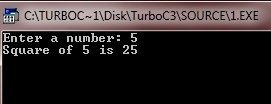In the above C program, the value of the argument to square(), 5 (entered by the user), is copied into the parameter a. When the assignment, a=a*a takes place, this only modify the local variable a. The variable num, used to call the function square(), still has the value 5. Hence, the output is "Square of 5 is 25" as shown in the above output.

### C call by reference Example

Here is an example program which illustrates the concept of call by reference in C language. Here we have performed the swapping of two numbers using the function, just concentrate on this.

```/* C Functins - Call by Reference Program */

#include<stdio.h>
#include<conio.h>

void swap(int *a, int *b);

void main()
{
int num1, num2;
clrscr();

printf("Enter the two number: ");
scanf("%d%d", &num1, &num2);

printf("\nValues before swapping:\n");
printf("num1 = %d \t num2 = %d\n", num1, num2);

swap(&num1, &num2);        // pass the address of num1 and num2

printf("\nValues after swapping:\n");
printf("num1 = %d \t num2 = %d\n", num1, num2);

getch();
}

void swap(int *a, int *b)
{
int temp;

temp = *a;    // save the value at address a
*a = *b;
*b = temp;

printf("\nValues in the function swap() after swapping:\n");
printf("num1 = %d \t num2 = %d\n", *a, *b);
}```

Here is the sample output of the above C program: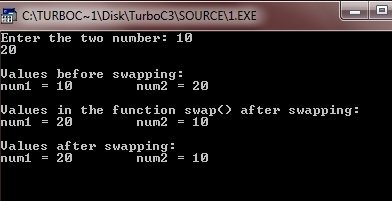In the above program, the variable num1 is assigned the value 10 (entered by the user), and the variable num2 is assigned the value 20 (entered by the user). Then the function swap() is called with the addresses of num1 and num2. And the unary operator &, is used to produce the address of the variable. Therefore, the addresses of num1 and num2, not their values, are passed into the function swap(). So the changes made in the function swap(), will change the actual value of the variables num1 and num2.

## Calling Functions with Arrays in C

When an array is used as a function argument, then its address is passed to a function. Here is an example program. In this program, we created a function print_in_upper(), which simply prints its string argument into uppercase:

```/* C Functions - Calling Functions with Arrays */

#include<stdio.h>
#include<ctype.h>
#include<conio.h>

void print_in_upper(char *string);

void main()
{
char str;
clrscr();

printf("Enter a string: ");
gets(str);

print_in_upper(str);

printf("\nThe string is now in uppercase: ");
printf("%s", str);

getch();
}

void print_in_upper(char *string)
{
register int i;

for(i=0; string[i]; i++)
{
string[i] = toupper(string[i]);
putchar(string[i]);
}
}```

Here is the sample run of this C program: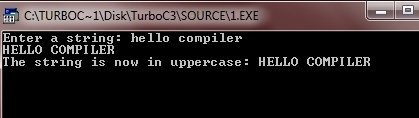You can make the same program more attractive like this:

```/* C Functions - Calling Functions with Arrays */

#include<stdio.h>
#include<ctype.h>
#include<conio.h>

void print_in_upper(char *string);

void main()
{
char str;
int i;
long int l;
clrscr();

printf("Enter a string: ");
gets(str);

printf("Converting string into uppercase");
for(i=0; i<3; i++)
{
for(l=0; l<50000000; l++)
{
}
printf(".");
}
printf("\n");

print_in_upper(str);

printf("\nThe string is converted into uppercase successfully\n");
printf("%s", str);

getch();
}

void print_in_upper(char *string)
{
register int i;

for(i=0; string[i]; i++)
{
string[i] = toupper(string[i]);
putchar(string[i]);
}
}```

Here is the sample run of the above C program: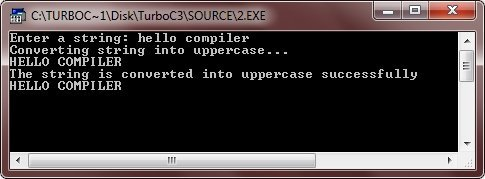## C Function Example

Here is an example program of C function:

```/* C Functions - Example Program */

#include<stdio.h>
#include<conio.h>

int sum(int, int);
int subtract(int, int);
int multiply(int, int);
int divide(int, int);

void main()
{
int num1, num2;
clrscr();

printf("Enter the two number: ");
scanf("%d%d", &num1, &num2);

printf("\nSummation of %d and %d is %d", num1, num2, sum(num1, num2));
printf("\nSubtraction of %d and %d is %d", num1, num2, subtract(num1, num2));
printf("\nMultiplication of %d and %d is %d", num1, num2, multiply(num1, num2));
printf("\nDivision of %d and %d is %d", num1, num2, divide(num1, num2));

getch();
}

int sum(int a, int b)
{
return a+b;
}
int subtract(int a, int b)
{
return a-b;
}
int multiply(int a, int b)
{
return a*b;
}
int divide(int a, int b)
{
return a/b;
}```

Here is the sample run of the above C function example program: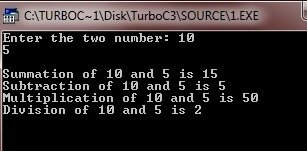Tools
Calculator